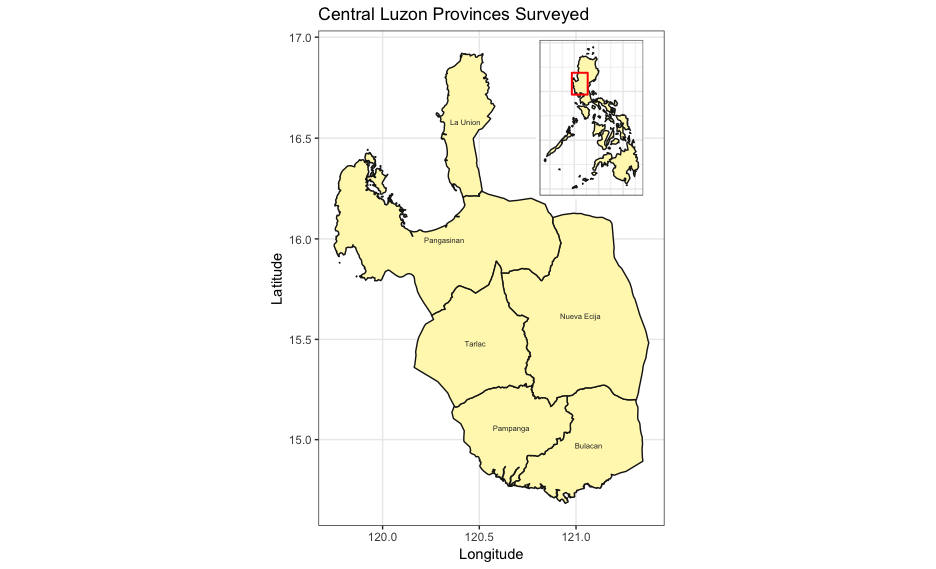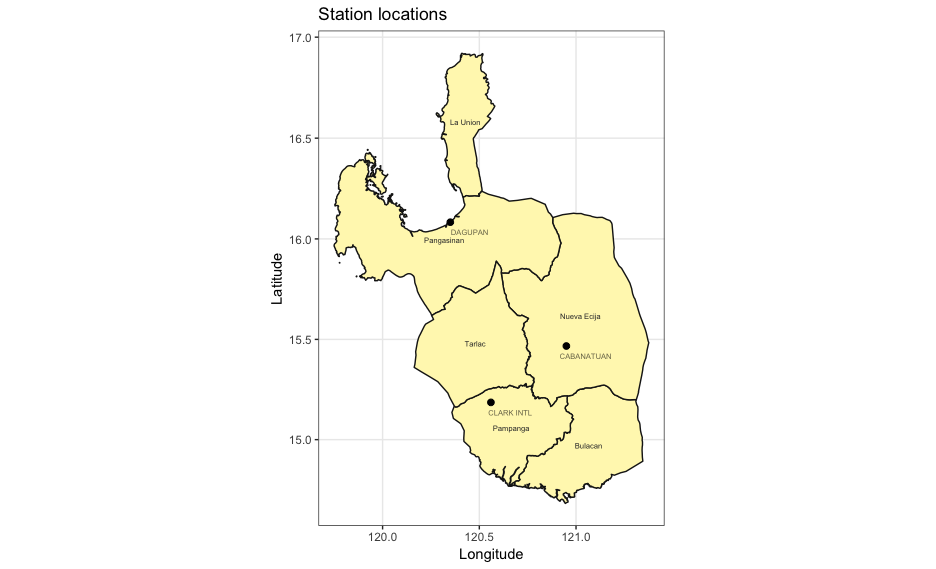## Purpose

The IRRI (International Rice Research Institute) survey loop in Central Luzon is a study that aims to monitor the changes in rice farming in the major rice producing area of the Philippines - the Central Luzon region, which is called as the “rice bowl of the Philippines”. Data have been collected in this project since the 1960s. See, http://ricestat.irri.org/fhsd/php/panel.php?page=1&sortBy=title&sortOrder=ascending# for the panel data.

This vignette details how to find and retrieve weather data for the area that this survey covers for the time period of 1960-2016. Methods that are detailed include:

• retrieving a spatial object of provincial level data;

• sub-setting this data for the provinces of interest;

• finding stations that fall within this polygon;

• determining which stations provide data for the specified time-period, 1960-2015; and

## Retrieve PHL provincial map and select loop provinces

As a first step, we’ll use the raster package to retrieve data from GADM that will provide the provincial spatial data for the survey area. We will then use this to find the stations that fall within the provinces where the study was conducted.

Using raster::getData() fetch level 0 (national) and 1 (provincial) data for the Philippines.

library(raster)
RP0 <- getData(country = "Philippines", level = 0)
RP1 <- getData(country = "Philippines", level = 1)

Now we will select the provinces involved in the survey and make a new object called Central_Luzon from the provincial level data, RP1.

Central_Luzon <- RP1[RP1@data$NAME_1 == "Pampanga" | RP1@data$NAME_1 == "Tarlac" |
RP1@data$NAME_1 == "Pangasinan" | RP1@data$NAME_1 == "La Union" |
RP1@data$NAME_1 == "Nueva Ecija" | RP1@data$NAME_1 == "Bulacan", ]

Next, create a map inset showing where the Central Luzon Loop survey takes place.

First we’ll use gSimplify() from rgeos to simplify the map of the Philippines to make the map generation in the next few steps much quicker.

library(rgeos)
RP0 <- gSimplify(RP0, tol = 0.05)
library(ggplot2)
library(grid)
library(gridExtra)
library(sp)

# get center coordinates of provinces in Central Luzon
CL_names <- data.frame(coordinates(Central_Luzon))

# this is then used to label the procinces on the map
CL_names$label <- Central_Luzon@data$NAME_1

# Main map
p1 <- ggplot() +
geom_polygon(data = Central_Luzon,
aes(x = long,
y = lat,
group = group),
colour = "grey10",
fill = "#fff7bc") +
geom_text(data = CL_names, aes(x = X1,
y = X2,
label = label),
size = 2,
colour = "grey20") +
theme(axis.text.y = element_text(angle = 90,
hjust = 0.5)) +
ggtitle("Central Luzon Provinces Surveyed") +
theme_bw() +
xlab("Longitude") +
ylab("Latitude") +
coord_map()

# Inset map
p2 <- ggplot() +
geom_polygon(data = RP0, aes(long, lat, group = group),
colour = "grey10",
fill = "#fff7bc") +
coord_equal() +
theme_bw() +
labs(x = NULL, y = NULL) +
geom_rect(aes(xmin = extent(Central_Luzon),
xmax = extent(Central_Luzon),
ymin = extent(Central_Luzon),
ymax = extent(Central_Luzon)),
alpha = 0,
colour = "red",
size = 0.7,
linetype = 1) +
theme(axis.text.x = element_blank(),
axis.text.y = element_blank(),
axis.ticks = element_blank(),
axis.title.x = element_blank(),
axis.title.y = element_blank(),
plot.margin = unit(c(0, 0, 0 ,0), "mm"))

grid.newpage()
# plot area for the main map
v1 <- viewport(width = 1, height = 1, x = 0.5, y = 0.5)

# plot area for the inset map
v2 <- viewport(width = 0.28, height = 0.28, x = 0.67, y = 0.79)

# print the map object
print(p1, vp = v1)
print(p2, vp = v2)Figure 1: Station locations

## Identify stations in area of interest

Next, make a list of stations that are within this area. GSODR provides a table of the metadata associated with GSOD stations, which includes latitude and longitude that can be used to identify stations falling within the area of interest.

library(GSODR)

# load the station metadata file from GSODR (this loads isd_history in your
# R sesion)

isd_history <- as.data.frame(isd_history)

# convert to a spatial object to find stations within the states
coordinates(isd_history) <- ~ LON + LAT
proj4string(isd_history) <- proj4string(Central_Luzon)

# what are the coordinates? We use the row numbers from this to match the
# stations data.frame
station_coords <- coordinates(isd_history[Central_Luzon, ])

# get row numbers as an object
rows <- as.numeric(row.names(station_coords))

# create a data frame of only the stations which rows have been identified
loop_stations <- as.data.frame(isd_history)[rows, ]

# subset stations that match our criteria for years
loop_stations <- loop_stations[loop_stations$BEGIN <= 19600101 & loop_stations$END >= 20151231, ]

print(loop_stations[, c(1:2, 3, 7:12)])
        USAF  WBAN   STN_NAME    LAT    LON ELEV_M    BEGIN      END        STNID
25617 983250 99999    DAGUPAN 16.083 120.35    2.0 19450119 20180610 983250-99999
25619 983270 99999 CLARK INTL 15.186 120.56  147.5 19450214 20180610 983270-99999
25623 983300 99999 CABANATUAN 15.467 120.95   32.0 19490101 20180610 983300-99999

These are all of the stations that are available within the provinces of interest and the locations in the provinces.

### Map station locations

p1 +
geom_point(data = loop_stations,
aes(x = LON,
y = LAT),
size = 2) +
geom_text(data = loop_stations,
aes(x = LON,
y = LAT,
label = STN_NAME),
alpha = 0.6,
size = 2,
position = position_nudge(0.1, -0.05)) +
ggtitle("Station locations")

## Import the weather data into R

### Use get_GSOD() to fetch the requested station files

This example shows how you could construct a query using the get_GSOD() function. Be aware that it may result in incomplete data and error from the server if it stops responding. We’ve done our best to make GSODR handle these errors, but if it does this, see the following option for using the reformat_GSOD() function.

PHL <- get_GSOD(station = loop_stations[, 12], years = 1960:2015)

### Another option, use reformat_GSOD()

GSODR provides a function for dealing with local files that have been transferred from the server already as well, reformat_GSOD(). If the previous example with get_GSOD() does not work, this is a good alternative that takes a bit more intervention but gives the same results.

Using your FTP client (e.g., FileZilla) log into the NCEI FTP server, <ftp.ncdc.noaa.gov> and navigate to /pub/data/gsod/. Manually downloading the files for each station listed above from 1960 to 2015 is possible, but tedious. An easier solution is to simply download the annual files found in each yearly directory, “gsod-YYYY.tar” and untar them locally and then use R to list the available files and select only the files for the stations of interest. Lastly, write the data to disk as a CSV file for saving and later use.

years <- 1960:2015

loop_stations <- eval(parse(text = loop_stations[, 12]))

# create file list
loop_stations <- do.call(
paste0, c(expand.grid(loop_stations, "-", years, ".op.gz"))
)

local_files <- list.files(path = "./GSOD", full.names = TRUE, recursive = TRUE)
local_files <- local_files[basename(local_files) %in% loop_stations]

loop_data <- reformat_GSOD(file_list = local_files)

readr::write_csv(loop_data, path = "Loop_Survey_Weather_1960-2015", path = "./")Figure 2: Station locations